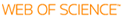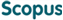Cited time inCited time inAnalysis and Modeling of Capacity Fading in Lithium Ion Batteries

Title
Analysis and Modeling of Capacity Fading in Lithium Ion Batteries
Author(s)
Appiah, Williams Agyei
DGIST Authors
Appiah, Williams AgyeiLee, Yong MinRyou, Myung-Hyun
이용민
Ryou, Myung-Hyun
Issued Date
2020
Awarded Date
2020/08
Type
Thesis
Description
Lithium ion batteries, Capacity fade, Physics-based model, Physico chemical model, Chemo-mechanical model.
Abstract
This thesis presents a non-destructive capacity-fading analysis method to identify the various degradation mechanisms of high energy density active materials for Li ion cells. The key objective of this method is the extraction of information on degradation from physics-based model parameters that changes with cycling via a parameter estimation technique.

List of figures ……………………………………………………………… …………xii

List of tables …………………………………………………………….…….… …. xvi

Nomenclature …………………………………………………………………………xvii

1 Introduction and literature review 1
1.1 Introduction 1
1.2 Post-mortem analysis methods 8
1.2.1 Surface sensitive chemical analysis methods 9
1.2.2 Bulk electrode chemical analysis methods 11
1.2.3 Electrolyte analysis methods 12
1.3 Battery performance-based analysis methods 14
1.3.1 Electrochemical voltage spectroscopy 14
1.3.2 Identification and tracking of model parameters 17
1.4.1 Empirical modeling method 20
1.4.2 Physics-based models 24
1.5 Focus and objectives 30
1.6 Outline 32

2 Capacity fade analysis of spinel-based cathode materials 34
2.1 Introduction 34
2.2 Experiment 36
2.3 Results and discussion 37
2.3.1 Parameter Estimation 37
2.3.2 Model prediction 41
2.3.3 Analysis of capacity fade 45
2.4 Conclusion 47

3 A capacity fade model for spinel-based cathode materials 48
3.1 Introduction 48
3.2. Model development 50
3.2.1 Modeling of Mn2+ dissolution in the cathode 50
3.2.2 Modeling of CEI formation in the cathode 52
3.2.3 Modeling of the SEI and Mn side reactions at the anode 54
3.3. Parameter estimation 58
3.4. Results and discussion 59
3.5. Conclusion 69

4 Application of capacity fade model: Accelerated cyclic aging analysis 70
4.1 Introduction 70
4.2 Methodology 72
4.2.1 Experimental data collection 73
4.2.2 Simple empirical life model (SELM) development 74
4.3 Results and discussion 74
4.4 Conclusion 83

5 Capacity fade analysis of anode materials with huge volume expansion 84
5.1 Introduction 84
5.2 Experiment 86
5.2.1 Treatment of Cu current collector with Polydopamine 86
5.2.2 Preparation of Electrode 86
5.2.3 Assembling of cell 86
5.2.4 Measurement of electrochemical performance 87
5.3 Results and discussion 87
5.3.1 Parameter Estimation 87
5.3.2 Model Predictions 91
5.4 Conclusion 102

6 A chemo-mechanical degradation model 103
6.1 Introduction 103
6.2 Model development 104
6.2.1 Modeling of SEI formation 105
6.2.2 Modeling of contact resistance 110
6.2.3 Modeling of particle isolation 114
6.2.4 Modeling of Li ions inventory 115
6.2.5 Modeling the effect of the PD interlayer 117
6.2.6 Coupling between lithiation kinetics and mechanical stress 118
6. 3 Pseudo-two-Dimensional (P2D) model – Incorporation 120
6. 4 Results and discussion 121
6.4.1 Model validation 121
6.4.2 Simulation results 123
6. 5 Conclusion 129

7 Application of chemo mechanical model 130
7.1 Introduction 130
7.2 Experiment 132
7.3 Results and discussion 133
7.3.1 Experimental results 133
7.3.2 Model validation 135
7.3.3 Simulation results 137
7.4 Conclusion 145

8 Conclusion and future work 146
8.1 Contributions 146
8.1.1 Multiphysics-based model capacity fade analysis 147
8.1.2 A capacity fade model for spinel-based cathode materials 148
8.1.3 A time effective cyclic accelerated aging analysis framework 148
8.1.4 A chemo mechanical degradation model 149
8.1.5 Practical relevance 150
8.2 Future work 152
8.2.1 Modeling of Ni-rich cathode materials 152
8.2.2 Exploring the negative side of adhesive thin film interlayers 152
8.2.3 Investigation into degradation mechanisms of large format Li ion cells 153
8.2.4 Short term future research 153
References 158

Appendix A 174
A.1 Model development: SEI formation at cathode 174
A.2 Transport equations 175

Appendix B 179
B.1 Expressions 179

Appendix C 181
C.1 Li ions inventory in Si electrode 181
C.2 Effect of polydopamine design on degradation parameters 181

List of figures

1.1. Ragonne plot of various cell chemistries 2
1.2. Specific energy density from pack to materials level 3
1.3. Degradation mechanism of Si anodes 5
1.4. Layered-to-spinel transformation of Ni-rich cathode materials 6
1.5. Correlation between (a) voltage profile and (b) IC and (c) DV 15
1.6. Degradation mechanisms in Li-ion cells 19
1.7. Schematic diagram of Li ion battery P2D model 25
1.8. Schematic diagram of single particle model (SPM) 28
2.1. Discharge capacity retention of LiMn2O4/graphite cells at 25 and 60 °C. 37
2.2. Comparison of experimental discharge profiles and model-prediction 38
2.3. Changes in degradation parameters of LiMn2O4/graphite cells 40
2.4. Predicted SOC at the EOD at (a) 25 °C and (b) 60 °C 41
2.5. Extrapolation of the model parameters 42
2.6. Physics-based and empirical model prediction at (a) 25 and (b) 60 °C 43
2.7. The predicted SOCs for the positive and negative electrode at the EOD 44
3.1. Physico-chemical degradation model best fit of experimental data 60
3.2. Correlation between Li ion transport and SOC at 25 °C and 60 °C 62
3.3. Concentration profile of the solvent species at the film/electrode interphase 63
3.4. Film resistance at the end of the discharge in the cathode and anode 64
3.5. Relative volume fraction of the active cathode material at 25 ºC and 60 ºC 65
3.6. (a) Changes in the cell capacity retention at different cut off volatges and,
(b) Model best-fit to experimental data . 66
3.7. Cycle performance at different discharge rates . 67
4.1. Summary of proposed accelerated cyclic aging analysis framework 73
4.2. Experimental results of discharge-capacity retention of LiMn2O4/graphite
cells cycled at temperatures of 25 and 60 ºC 75
4.3. Physics-based model best fit to experimental data 76
4.4. Simulated (a) cycling performance, (b) diffusion coefficient constant of
the cathode, (c) cathode electrolyte interphase (CEI) resistance and (d)
solid electrolyte interphase (SEI) resistance, at various temperatures 78
4.5. Simulations using SELM and PCM–PCEM at different temperatures. 79
4.6. Dependence of (a) capacity-fade constant, and (b) power-law factor on
temperature.. 80
4.7. Qualitative analysis of electrochemical voltage spectroscopy . 81
4.8. Predicted number of cycles at different temperatures as a function of………..83
5.1. Comparison of experimental discharge profiles and model predictions. 88
5.2. The changes of model parameters with cycling 90
5.3. The simulated SOCs for the Si/Li half-cells. 91
5.4. The extrapolation of the physics-based P2D model parameters 92
5.5. Comparison of physics-based and empirical model predictions 93
5.6. The predicted SOCs for the bare and PD-treated Cu current collectors 94
5.7. The formation mechanism of EMDOHC and LiEDC 96
5.8. The percentage of Li ion loss in Si/Li cells 98
5.9. The net loss of Li ions in Si/Li cells 99
5.11. Schematic diagram showing the effect of the polydopamine interlayer on
the number of isolated particles after several cycles.. 101
6.1. Degradation mechanisms of Si electrode with PD-interlayer between the
Cu current collector and the composite electrode. 105
6.2. Block diagram of Li ions inventory in the Si electrode 115
6.3. Schematic diagram of the cross section of the cell modeled in this study 120
6.4. Chemo-mechanical degradation model best of experimental data. 123
6.5. Changes in the film resistance and the surface area. 124
6.6. Correlation between the electron transfer rate constant and number of
cycles for the PD-treated and bare Cu current collector. 125
6.7. Changes in (a) initial SOC, (b) SOC at EOC and Simulated fractional
Li ion loss in Li/Si cells 126
6.8. Relative contribution of various degradation mechanisms 128
7.1. Schematic diagram of cell designs used in this study 133
7.2. Experimental rate performance and the Peukert coefficient of the Li/Si 134
7.3. Experimental and simulation voltage profiles of the three cells 137
7.4. Simulated salt concentration profiles across the Si composite electrode . 138
7.5. Simulated Li ion concentration in the solid phase across the Si composite 139
7.6. (a) Contact resistance and (b) Adhesive strength . 141
7.7. Specific capacity as a function of cell design parameters. 142
7.8. Effect of PD film design parameters on capacity retention 144
C.1. Li ions inventory in the Si composite electrode . 181
C.2. Effect of adhesion strength between Si composite electron an Cu current
collector on (a) contact resistance and (b) Li ions loss to isolation. 181
C.3. Effect of various PD film (a) thickness (coverage = 1), (b) thickness
(coverage = 0.8) on the rate constant. 182
C.4. Effect of various PD film coverage on the reaction rate constant. 182
C.5. Effect of PD film coverage on (a) contact resistance and (b) Li ions loss
to isolation. 183

List of tables

1.1. The governing equations of P2D model 26
2.1. Design parameters, used in this study. 38
2.2. Mathematical expression used for the extrapolation in Figure 2.5 41
2.3. Empirical model expressions 44
3.1. Model parameters. 58
4.1. Equations for predicting the accelerated capacity-fade 78
5.1. Model parameters used in this study 88
5.2. Empirical model expressions and parameters 93
6.1. Table of parameters used for the model prediction. 121
URI
http://dgist.dcollection.net/common/orgView/200000321089

http://hdl.handle.net/20.500.11750/12153
DOI
10.22677/thesis.200000321089
Degree
Doctor
Department
Department of Energy Science and Engineering
Publisher
DGIST
Related Researcher
•Lee, Yong Min 에너지공학과
• Research Interests Battery; Electrode; Electrolyte; Separator; Simulation
Files in This Item:200000321089.pdf